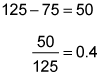##### ACT: 1,001 Practice Questions For DummiesIf you want a high percentage score on the ACT Math exam, then you should know how to solve percentage problems. As you'll see in the following practice questions, percentages appear in a wide range of problems, from business math to algebra.

## Practice questions

1. Given that 6 percent of (a + b) = 12 percent of b, which of the following must be true?

A. a < b B. a > b C. a = b D. a + b = 0 E. a < 0 and b < 0

2. The cost of a tablet increased 25 percent from 2014 to 2015. In 2016, the cost of the tablet was 1/4 less than its 2014 cost. By what percentage did the cost of the tablet decrease from 2015 to 2016?

A. 5% B. 25% C. 40% D. 50% E. 75%

1. The correct answer is Choice (C).

An easy way to deal with percentages is to use the number 100. In this case, say that (a + b) = 100. Then 6 percent of 100 = 6. That means that 12 percent of b is 6. Solve for b:

If a + b = 100, then a = 50 and a = b.

2. The correct answer is Choice (C). Give this problem a real value to work with. Say the 2014 price of the tablet is \$100. If the price increased 25 percent between 2014 and 2015, its 2015 price is \$125. The next year the price was 1/4 less its 2014 price of \$100, which means it cost \$75 in 2016. To find the percent decrease between 2015 and 2016, subtract the two prices and divide the difference by the 2015 price:The percent decrease from 2015 to 2016 is 40 percent.